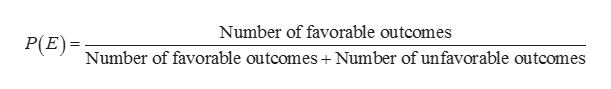Compute the probability of an event E if the odds in favor of E are 2/5.

Question

Compute the probability of an event E if the odds in favor of E are 2/5.

Step 1

Odds of an event:

The odds an event is given by the ratio of the probability of the event to its probability of compliment of the event. That is, the odds of an event E is given below.help_outlineImage TranscriptioncloseOdds of an event = Number of favorable outcomes Number of unfavorable outcomes fullscreen
Step 2

Probability of an event:

The probability of an event is the number of favourable outcomes divided by the sum of number of favourable and unfavourable outcomes.help_outlineImage TranscriptioncloseNumber of favorable outcomes P(P Number of favorable outcomes + Number of unfavorable outcomes )= fullscreen
Step 3

Computation of probability of an event E:

It is given that odds in favour of E is 2/5.

That is, number of favourable outcomes is 2.

The number of unfavourable outcomes is 5.

By substituting the va...

Want to see the full answer?

See Solution

Want to see this answer and more?

Our solutions are written by experts, many with advanced degrees, and available 24/7

See Solution
Tagged in

Other